International Journal of Fluid Mechanics & Thermal Sciences
Volume 1, Issue 1, April 2015, Pages: 8-13

Time Step Size Effect on the Liquid Sloshing Phenomena

Abdallah Bouabidi, Zied Driss, Mohamed Salah Abid

Laboratory of Electro-Mechanic Systems (LASEM), National School of Engineers of Sfax (ENIS), University of Sfax, Sfax, Tunisia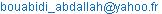(A. Bouabidi)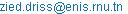(Z. Driss)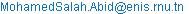(M. S. Abid)

Abdallah Bouabidi, Zied Driss, Mohamed Salah Abid. Time Step Size Effect on the Liquid Sloshing Phenomena. International Journal of Fluid Mechanics & Thermal Sciences. Vol. 1, No. 1, 2015, pp. 8-13. doi: 10.11648/j.ijfmts.20150101.12

Abstract: In this paper, the effect of the time step size on the numerical results of the liquid sloshing problem was studied for a laterally moving three-dimensional (3D) rectangular tank in a battery cell. The commercial software "Fluent" has been used to predict the local flow characteristics in the tank. To simulate 3D incompressible viscous two phase ﬂow in a tank, partially ﬁlled with liquid, the volume of ﬂuid (VOF) method based on the ﬁnite volume method has been considered. The comparison between numerical and experimental results confirms the validity of the numerical method.

Keywords: Fluid Dynamics, Turbulent Flow, Volume of Fluid, Liquid Sloshing

1. Introduction

Lateral sloshing flow is defined as a time dependent fluid motion with free surface in a container oscillating periodically along the horizontal direction perpendicular to the gravity. This subject has been approached by many authors from different angles. Cho and Lee  carried out a parametric investigation on the two-dimensional nonlinear liquid sloshing in baffled tank under horizontal forced excitation based on the fully nonlinear potential flow theory. Gavrilyuk  analyzed the linear and nonlinear fluid sloshing problems in a circular conical tank in a curvilinear coordinate system. The linear sloshing modes are approximated by a series of the solid spheric harmonics. Chen et al.  developed a finite-difference method to analyse sloshing in tanks. Akyildiz et al.  investigated both experimentally and numerically the sloshing moment amplitudes in a rectangular tank for a wide range of rolling frequencies. Gavrilyuk et al.  investigated analytically the relationship between the frequency of the sloshing, the width of the baffle and the vertical position of the baffle within the cylindrical tank. Biswal et al.  studied the 2D nonlinear sloshing in both cylindrical and rectangular tanks with rigid baffles using FEM. Baffles placed close to the free surface of the fluid in the tank were found more effective in reducing the effect of sloshing than baffles above the free surface. Souto-Iglesias et al.  investigated both experimentally and numerically the sloshing moment amplitudes in a rectangular tank for a wide range of rolling frequencies. Kim et al.  studied the coupling effects between the ship motion and sloshing flows using the impulse–response–function formulation for linear ship motion and a computational fluid dynamics (CFD) simulation for nonlinear sloshing flows. Nasar et al.  analyzed experimentally the sloshing phenomena of liquid in partially filled tanks mounted on a barge exposed to regular beam waves. Maleki and Ziyaeifar  analysed hydrodynamic damping ratio of liquid sloshing in baffled tanks undergoing horizontal excitation analytically using the Laplace’s differential equation solution, carried a series of experiments employing a tank model on a shake-table, and showed that the ring baffles were more effective in reducing the sloshing oscillations. Dongming et al.  developed a numerical model to study three-dimensional (3D) liquid sloshing in a tank with baffles. The numerical model solves the spatially averaged Navier–Stokes equations, which are constructed on a non-inertial reference frame having six degree-of-freedom (DOF) of motions. Panigrahy et al.  showed experimentally that baffles in a tank decrease the sloshing effect considerably because sharp- edged baffles create turbulence in the flow field thereby dissipating the excess kinetic energy to the walls. Abadi et al.  used the boundary element method to determine the frequencies and mode shapes of liquid sloshing in 3-D baffled containers with arbitrary geometries. Pal and Bhattacharyya studied 3-D liquid sloshing in a tank with a horizontal baffle and a vertical baffle, respectively, and pointed out that a vertical baffle is more effective on reducing the sloshing amplitude. Godderidge et al.  presented the multiphase CFD modeling of a lateral sloshing tank. Nasar et al.  investigated the interaction between the liquid sloshing in a rectangular tank equipped inside the barge and the barge responses through a comprehensive experimental program. Belakroum et al.  predicted the damping effect of baffles on sloshing in tanks partially filled with liquid. Huang et al.  studied experimentally sloshing phenomena in a rectangular tank under a sway excitation. Thiagarajan et al.  present the rudiments of a linear potential theory for sloshing motions in a two-dimensional rectangular tank, due to small amplitude sway motions. Xue and Lin  developed a three-dimensional (3-D) numerical model to study viscous liquid sloshing in a tank with internal baffles of different shapes and arrangements. Wu et al.  used a time-independent finite difference scheme with fictitious cell technique to study viscous fluid sloshing in 2D tanks with baffles. Akyildiz  presented a numerical algorithm based on the volume of fluid (VOF) technique to study the non linear behaviour of liquid sloshing. Jung et al.  investigated the effect of the vertical baffle height on the liquid sloshing in a laterally moving three-dimensional (3D) rectangular tank. To simulate 3D incompressible viscous two phase flow in a tank partially filled with liquid, they used the volume of fluid (VOF) method based on the finite volume method. Gavrilyuk et al.  studied the sloshing phenomena of an ideal incompressible liquid in a rigid truncated conical tank when the tank performs small-magnitude oscillatory motions with the forcing frequency close to the lowest natural sloshing frequency. Akyıldız  studied experimentally the liquid sloshing in a cylindrical tank with various ﬁll levels and ring bafﬂes under the excitations. Bouabidi et al.  studied the effect of the vertical baffle height on liquid sloshing in an accelerating rectangular tank.

Despites the numerous papers already devoted to this subject, there are still important questions to be answered. It is of interest to focus on the effect of time step size on the accuracy of the numerical results. In this paper, we are interested in studying of the time step size effect on the numerical results of a liquid sloshing problem.

2. Geometrical System

Figure 1 shows the geometrical arrangement of a liquid in a 3D rectangular tank with the generation of rectangular meshes. The dimensions of the tank are 0.8 m in length (L), 0.5 m in height (H) and 0.4 m in width (b). For all the simulations in this study, the water depth or filling level (h) is assumed equal 70% of the tank height. The tank motion is the pure surge (translation in the x direction only).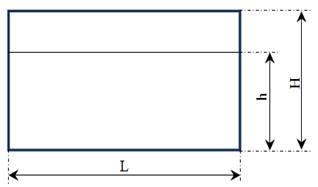Figure 1. Geometrical arrangement.

3. Numerical Model

The commercial CFD code "Fluent" has been used to present the local flow characteristics in the tank. The Navier-Stokes equations in conjunction with the standard k-ε turbulence model were solved using a finite volume discretization method [25-27].

3.1. Mathematical Model

The Navier-Stokes equations can be written in Cartesian form as: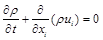(1)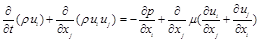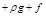(2)

where xi are Cartesian coordinates, ui are the corresponding velocity components, p is the pressure, ρ is the density, µ is the viscosity, g is the gravitational acceleration and f represents the external force due to the sinusoidal motion.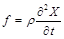(3)

The turbulence kinetic energy k and its rate of dissipation ε are obtained from the following transport equations: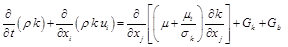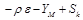(4)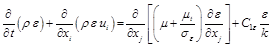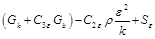(5)

Gk and Gb are the generation of turbulence kinetic energy (kg.m-1.s-3) respectively due to the mean velocity gradients and buoyancy. YM represents the contribution of the fluctuating dilatation in compressible turbulence to the overall dissipation rate. The turbulent viscosity μt (Pa.s) is given by: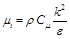(6)

The default values of the constants C, C, Cμ, σk and σε are presented in table 1.

Table 1. Model constants.

 C1ε C2ε Cμ σk σε 1.44 1.92 0.09 1.0 1.3

X represents the tank motion defined by the sinusoidal function as follow: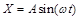(7)

Where A and ω are respectively the amplitude and the frequency. The amplitude is fixed for all simulation cases in this study. For a given rectangular prismatic tank, the natural frequencies of the fluid are given by: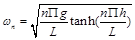(8)

Where L is the tank length, h is the water height and n is the mode number.The frequency of the sinusoidal motion in equation (8) has the same value for the lowest natural frequency of the fluid of the mode n=1 in equation (8). It was showed that for the problem of sloshing, the resonance frequency is different to the natural frequency of the fluid obtained from the equation for n=1.

The free surface is defined by the level-set function Ф defined as follow: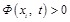, in air              (9)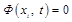, on surface           (10)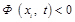, in water            (11)

The free surface deformation is described by the following equation: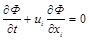(12)

3.2. Boundary Conditions and Meshing

The fluid boundaries in the problem considered are the container walls, bottom and the free surface of the fluid. On the container walls and bottom, the boundary condition is that the velocity of the flow at the wall is zero in the normal direction of the wall.No special treatment is required for the free surface due to the two-fluid flow model adopted herein. A no slip boundary condition is imposed by allowing the normal velocity to vanish at the wall: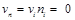(13)

Where ni is the unit vector normal to the wall.

The used mesh is presented in figure 2.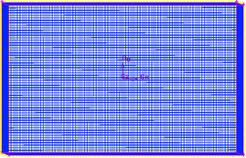Cells number Nx Ny Nz 19200 80 60 40

Figure 2. Generated mesh.

4. Comparison with Anterior Results

In figure 3, we show the time variations of the pressure at point defined by x=0.01, y=0.5 and z=0.2 for three different time step sizes. The numerical results reveal the weak dependence of the solutions on the considered time step size. Indeed, it has been noted that when using the second time step size (Tp/1000), a good agreement with the results of Chen et al.  is shown.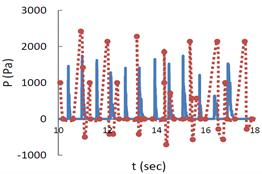(a) Tp/500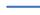Our model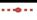Chen et al.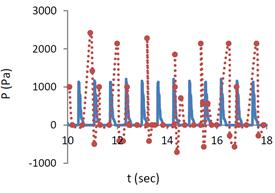(b) Tp/1000Our model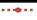Chen et al.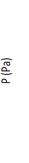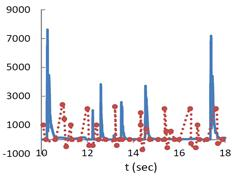(c) Tp/1500Our modelChen et al. 

Figure 3. Static pressure profile.

5. Numerical Results

The main aim of this section is to determine the behavior of the free surface elevation and the distribution of the pressure, the velocity fields, the vorticity, the turbulent viscosity, the turbulent dissipation rate, and the turbulent kinetic energy depending on the baffle height. The commercial CFD package "Fluent" is employed for all numerical predictions.

5.1. Free Surface Deformation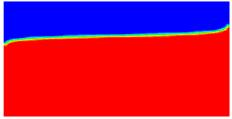(a) t=0.001 s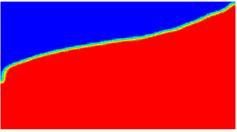(b) t=0.1s(c) t=0.8 s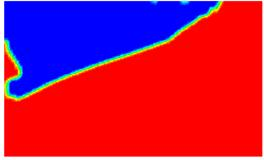(d) t=0.9 s

Figure 4. Free surface deformation.

Figure 4 presents the free surface deformationat different instances equal to t=0.001 s, t=0.1s, t=0.8s andt=0.9s.According to these results, it can be seen from there that the maximum free surface deformation is obtained at t=0.9 s. The results showed that the motion of the liquid becomes more important time. For the first instance, it has been observed that the motion of the liquid is very weak. For the second instance, at t=0.1 s the liquid sloshing becomes more important and the liquid reached the top of the tank

5.2. Hydrodynamic Parameters

Figure 5 displays the numerical results for the time step size Tp/1000. Particularly, we are interested on the velocity field, the magnitude velocity, the vorticity, the static pressure, the turbulent kinetic energy and the turbulent dissipation rate. According to these results, it has been noted that a recirculation zone appears in the top of the tank and the velocity is maximum at the free surface. For the static pressure, a compression zone is located in the down wall of the tank. The depression zone is located just in the top of the tank. The highest value of vorticity is located in the free surface and in the left side wall. The wake characteristic of the maximum values of the turbulent kinetic energy appears in the whole volume. However, near the wall the turbulent kinetic energy becomes very weak. The dissipation rate of the turbulent kinetic energy is maximal near the wall.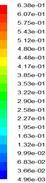(a)Velocity fields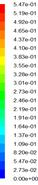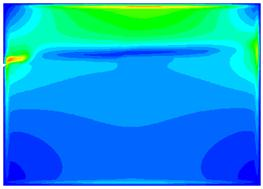(b)Magnitude velocity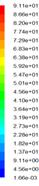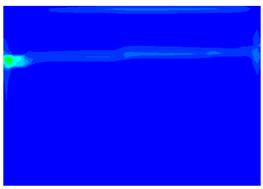(c)Vorticity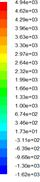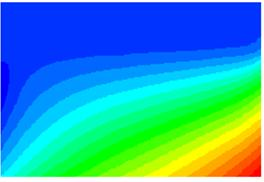(d)Static pressure(e)Turbulent kinetic energy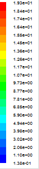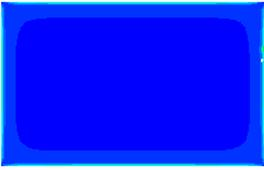(f)Dissipation rate of the turbulent kinetic energy

Figure 5. Local characteristics.

6. Conclusion

In this paper, we have studied the effect of the time step size on liquid sloshing phenomenon in a rectangulartank. The free surface deformation is presented at different instances. The hydrodynamic parameters describing the flow of liquid like the velocity fields, the static pressure and the turbulence characteristics in the unsteady state are also presented.A comparisonof the static pressure profile with anterior results is also presented. It appeared that the time step size strongly affects the numerical results. In the future, we propose to study sloshing phenomena in a battery cell for a micro-hybrid vehicle.

References

1. Cho, J.R., Lee, H.W., 2004, Numerical study on liquid sloshing in baffled tank by nonlinear finite element method. Comput. Methods Appl. Mech. Eng., 193(23-26), 2581-2598.
2. Gavrilyuk, I. P., Lukovsky, I. A. and Timokha, A.N., 2005, Linear and nonlinear sloshing in a circular conical tank, Fluid Dyn. Res., 37, 399-429.
3. Chen, B., Nokes, R., Roger, 2005, Time-independent finite difference analysis of fully non-linear and viscous fluid sloshing in a rectangular tank, Journal of Computational Physics, 209, 47-81.
4. Akyildiz, H., ErdemU¨nal, N., 2006, Sloshing in a three-dimensional rectangular tank: numerical simulation and experimental validation, Ocean Engineering 33, 2135-2149.
5. Gavrilyuk, I., Lukovsky, I., Trotsenko, Y., Timokha, A., 2006,Sloshing in a vertical cylindrical tank with an annular baffle. Part1. Linear fundamental solutions, J. Eng. Math., 54, 71-88.
6. Biswal, K.C., Bhattacharyya, S.K., Sinha, P.K., 2006, Non-linear sloshing in partially liquid filled containers with baffles, Int. J. Numer. Methods Eng.,68 , 317-337.
7. Souto-Iglesias, A., Delorme, L., Pérez-Rojas, L., Abril-Pérez, S., 2006, Liquid moment amplitude assessment in sloshing type problems with smooth particle hydrodynamics, Ocean Engineering, 33, 1462-1484.
8. Kim, Y., Nam, B.W., Kim, D.W., Kim, Y.S., 2007, Study on coupling effects of ship motion and sloshing. Ocean Eng., 34(16), 2176-2187.
9. Nasar, T., Sannasiraj, S.A. and Sundar, V., 2008, Experimental study of liquid sloshing dynamics in a barge carrying tank, Fluid Dyn. Res., 40(6), 427-458.
10. Maleki, A., Ziyaeifar, M., 2008, Sloshing damping in cylindrical liquid storage tanks with baffles, J. Sound Vib., 311(1), 372-385.
11. Liu,D., Lin, P., 2009, Three-dimensional liquid sloshing in a tank with bafﬂes, Ocean Engineering, 36(2), 202-212.
12. Panigrahy,P.K, Saha, P.K., Maity, U.K., 2009, Experimental studies on sloshing behavior due to horizontal movement of liquids in baffled tanks, Ocean Engineering, 36, 213-222.
13. Firouz-Abadi, R.D., Ghasemi, M., Haddadpour, H., 2011, A modal approach to second-order analysis of sloshing using boundary element method, Ocean Engineering, 38, 11-21.
14. Pal, P., Bhattacharyya, S.K., 2010,Sloshing in partially filled liquid containers-numerical and experimental study for 2-D problems, J. Sound Vib., 329, 4466-4485.
15. Godderidge, B., Turnock, S., Tan, M., Earl, C., 2009, An investigation of multiphase CFD modelling of a lateral sloshing tank,Comput Fluids, 38(1), 83-93.
16. Belakroum, R., Kadja, M., Mai,T.H., Maalouf, C., 2010, An efficient passive technique for reducing sloshing in rectangular tank partially filled with liquid, Mechanics Research Communications, 37, 341-346.
17. Huang, S., Duan,W.Y., Zhu, X., 2010, Time-domain simulation of tank sloshing pressure and experimental validation, J.Hydrodyn,22, 556-63.
18. Thiagarajann,K.P., Rakshit, D., Repalle, N., 2011, The air-water sloshing problem: Fundamental analysis and parametric studies on excitation and ﬁll levels, Ocean Engineering, 38, 498-508.
19. Xue, M.A., Lin, P., 2011, Numerical study of ring baffles effects on reducing violent liquid sloshing, Computers & Fluids, 52, 116-129
20. Wu,C.H., Faltinsen,O.M., Chen, B.F., 2012, Numerical study of liquids sloshing in tanks with baffles by time-independent finite difference and fictitious cell method, Computers & Fluids, 63, 9-26.
21. Akyildiz, H., 2012, A numerical study of the effects of the vertical baffle on liquid sloshing in two dimensional rectangular tanks, Journal of Sound and Vibration, 331, 41-52.
22. Jung, J.H., Yoon, H.S., Lee, C.Y., Shin, S.C., 2012, Effect of the vertical baffle height on the liquid sloshing in a three-dimensional rectangular tank, Ocean Engineering, 44, 79-89.
23. Gavrilyuk,I.P., Hermann, M., Lukovsky, I.A., Solodun, O.V., and Timokha, A.N., 2013,Weakly nonlinear sloshing in a truncated circular conical tank, Fluid Dyn. Res.,37, 399-429.
24. Akyıldız, H., ErdemUnal, N., Aksoy, H., 2013, An experimental investigation of the effects of the ring bafﬂes on liquid sloshing in a rigid cylindrical tank, Ocean Engineering, 59, 190-197.
25. Bouabidi, A., Driss, Z., Abid, M.S., 2013, Vertical Baffles Height Effect on Liquid Sloshing in an Accelerating Rectangular Tank, International Journal of Mechanics and Applications, 3(5), 105-116.
26. Driss Z., Abid, M.S., 2012, Use of the Navier-Stokes Equations to Study of the Flow Generated by Turbines Impellers, Navier-Stokes Equations: Properties, Description and Applications, Chapter 3, 51-138
27. Driss, Z., Ammar, M., Chtourou, W., Abid M.S., 2011, CFD Modelling of Stirred Tanks, Engineering Applications of Computational Fluid Dynamics, 1, Chapter 5, 145-258.

 Contents 1. 2. 3. 3.1. 3.2. 4. 5. 5.1. 5.2. 6.
Article ToolsAbstractPDF(2289K)